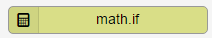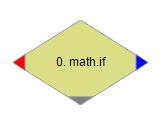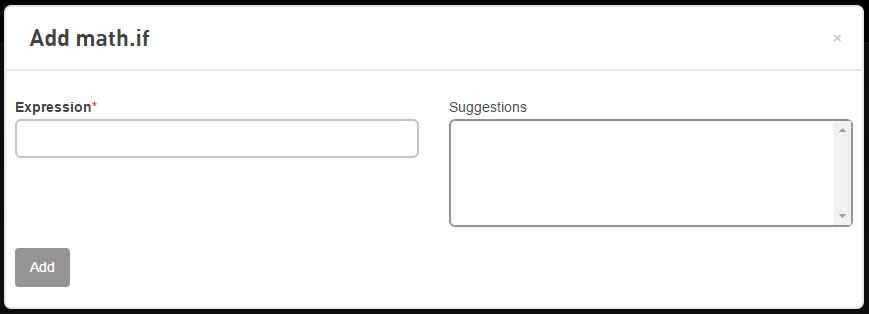# math.if action

This trigger action will perform a comparison using the expression provided to it. This trigger action allows for multiple logical action routes to be handled in one trigger.

## Action

This trigger action is found under the Math heading.## Canvas

When dragged onto the canvas, the appearance of the action is a diamond with three routing points.The left routing point (red) is the failure route. This route will be taken if the expression to be evaluated results in an error.

The bottom routing point (gray) is the false route. This route will be taken if the expression evaluates to false. For example: if the Expression is \$(event.prop.value) > 50 and \$(event.prop.value) contains the value 49.

The right routing point (blue) is the true route. This route will be taken if the expression evaluates to true. For example: if the Expression is \$(event.prop.value) > 50 and \$(event.prop.value) contains the value 51.

## Form## Parameter

Name Type Required Description
Expression String Yes This field will contain a formula that performs a comparison during the execution of this trigger. For example: \$(event.prop.value) < 100
Expression

Every expression consists of two or more operands, zero or more arithmetic operators, and one relational operator. Operands are values, such as 0, 1, 3.14, and 1234.5678; or variables like \$(event.prop.value) and \$(action.0.value). Arithmetic operators are symbols that represent particular mathematical actions.

In the expression "\$(event.prop.value) + \$(action.0.value) < 10"\$(event.prop.value)\$(action.0.value), and 10 are operands, + is an arithmetic operator, and < is a relational operator.

The following is a list of valid arithmetic operators.

Operator

Description

+

-

Subtraction

*

Multiplication

/

Division

^

Exponentiation (for example: 2^3 = 8)

% Modulo
@ Unary minus

( )

Force precedence of evaluation

The following is a list of valid relational operators:

Logical Operator

Description

==

Equal to

>

Greater than

>=

Greater than or equal to

<

Less than

<=

Less than or equal to

!=

Not equal to

## Action variable

Name Type Description
\$(action.X.value) Integer The result of evaluating the expression, where 0 (zero) is false and non-zero values are true.